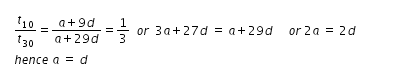# The sum of the first six terms oa an AP is 42.the ratio fo the 10th term

The sum of the first six terms oa an AP is 42.the ratio fo the 10th term to 30th term of an ap is 1/3.calculate the first term and the 13th term.

Let a be the first term and d be the common difference
Sum of 6 terms = S6 = (6/2) [ 2a + 5d ] = 42 or 2a+5d = 14 …(1)from eqn.(1), if we substitute a = d, then we get a = 2
13th term = 2+12(2) = 26